Open in App
Not now

# Frequency Distributions

• Last Updated : 28 Apr, 2021

Frequency Distribution occurs everywhere in our lives. Meteorological department, Data Scientists, Civil Engineers almost all the professions use frequency distributions in their professions. These distributions allow us to get insights from any data, see the trends, and predict the next values or the direction in which the data will go. There are two types of frequency distributions -grouped and ungrouped. Their usage depends on the data on which we are working. Their analysis is a really important part of probability and statistics. Let’s see these concepts in detail.

### Frequency Distributions

Frequency distributions tell us how frequencies are distributed over the values. That is how many values lie between different intervals. They give us an idea about the range where most of the values fall and the ranges where values are scarce.

A frequency distribution is an overview of all values of some variable and the number of times they occur.

Frequency distributions are of types:

1. Grouped Frequency Distributions- Values are divided between different intervals and then their frequencies are counted.
2. Un-Grouped Frequency Distributions- All distinct values of the variable are mentioned and their frequencies are counted.

Question: Let’s say we have data for the goals scored by a team in 10 different matches.

1, 0, 0, 3, 2, 0, 2, 3, 1, 1

Draw a frequency table to represent this data.

Solution:

Since there are less number of distinct values. We don’t have to group the data. We can just count the distinct values and their frequency.

This frequency table can also be represented in the form of a bar graph.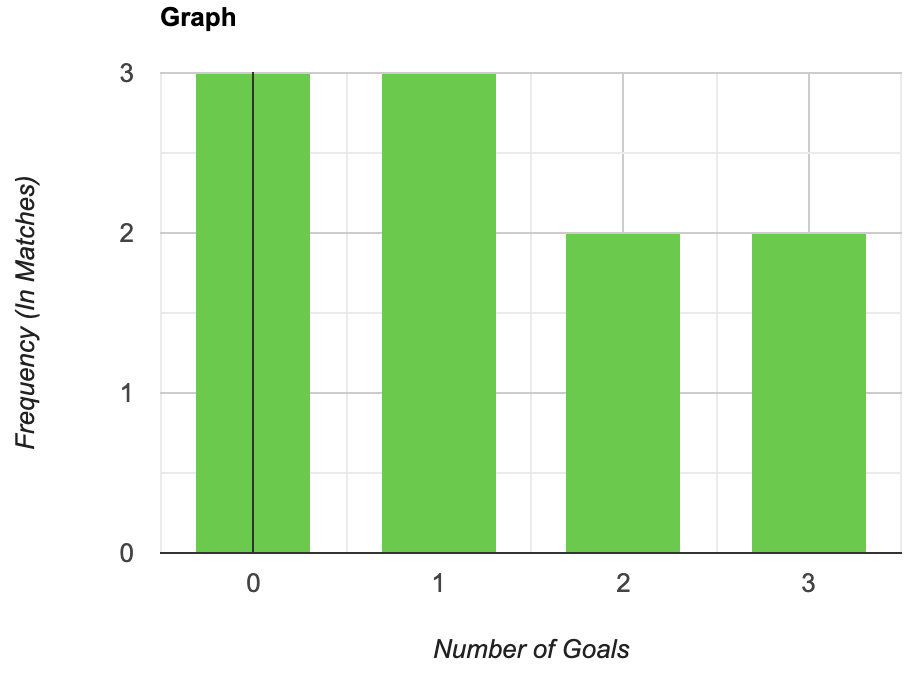A frequency distribution can also be represented by a line curve. The figure given below represents the line curve for the above problem.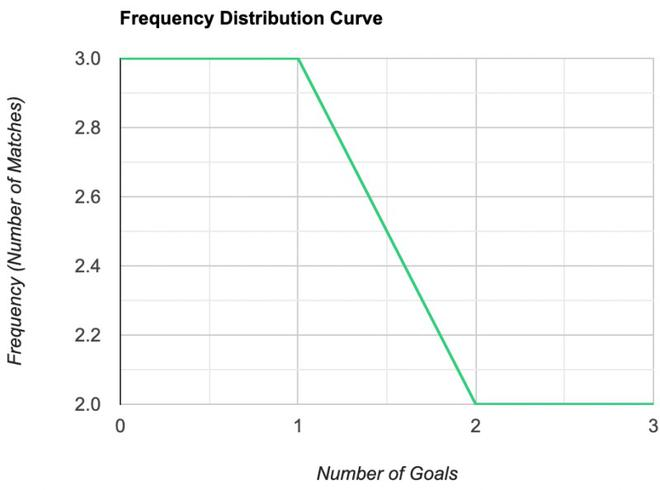Similarly, if there are a lot of distinct values, then we can group them and make grouped frequency distributions just like the previous case.

### Cumulative Frequency Distribution

Cumulative frequency is defined as the sum of all the frequencies in the previous values or intervals up to the current one. The frequency distributions which represent the frequency distributions using cumulative frequencies are called cumulative frequency distributions. There are two types of cumulative frequency distributions:

1. Less than type: We sum all the frequencies before the current interval.
2. More than type: We sum all the frequencies after the current interval.

Let’s see how to represent a cumulative frequency distribution through an example,

Question 1: The table below gives the values of runs scored by Virat Kohli in last 25 T-20 matches. Represent the data in the form of less than type cumulative frequency distribution:

Solution:

Since there are a lot of distinct values, we’ll express this in the form of grouped distributions with intervals like 0-10, 10-20 and so. First let’s represent the data in the form of grouped frequency distribution.

Now we will convert this frequency distribution into cumulative frequency distribution by summing up the values of current interval and all the previous intervals.

This table represents the cumulative frequency distribution.

Question 2: Represent the above the cumulative frequency distribution table in the form of cumulative frequency distribution line curve.

Solution:

To plot the line curve for the above table, use the mid-point of each interval and the corresponding value.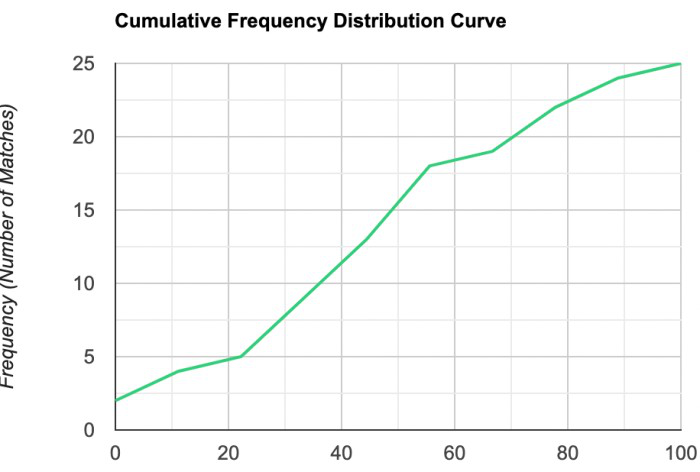### Coefficient of Variation

We know how to measure the dispersion of a series. We can use mean and standard deviation to describe the dispersion in the values. But sometimes while comparing the two series or frequency distributions becomes a little hard as sometimes both have different units.

For example: Let’s say we have two series, about the heights of students of a class. Now one series measures height in cm and the other one in meter. Ideally, both should have the same dispersion but the out methods of measuring the dispersion are dependent on units in which we are measuring. This makes such comparisons hard. For dealing with such problems, we define the Coefficient of Variation.

Coefficient of Variation is defined as,Here,andare the standard deviation and mean of the series.

The series having greater C.V. is said to be more variable than the other. The series having lesser C.V. is said to be more consistent than the other.

Comparing two frequency distributions with the same mean

We have two frequency distributions. Let’s sayandare the standard deviation and mean of the first series andandare the standard deviation and mean of the second series.

C.V of first series =C.V of second series =We are given that both series have same mean, i.eSo, now C.V for both series are,

C.V of first series =C.V of second series =Notice that now both series can be compared with the value of standard deviation only. Therefore, we can say that for two series with the same mean, the series with a larger deviation can be considered more variable than the other one.

Let’s see some examples of these concepts:

### Sample Problems

Question 1: Suppose we have a series, with a mean of 20 and variance is 100. Find out the Coefficient of Variation.

Solution:

We know the formula for Coefficient of Variation,Given mean= 20 and variance= 100.

Substituting the values in the formula,Question 2: Given two series with Coefficient of Variation 70 and 80. The means are 20 and 30. Find the values of standard deviation for both the series.

Solution:

In this question we need to apply the formula for CV and substitute the given values.

Standard Deviation of first series.Thus, the standard deviation of first series = 14.

Standard Deviation of second series.Thus, the standard deviation of first series = 24.

Question 3: Draw the frequency distribution table and frequency distribution curve for the following data:

2, 3, 1, 4, 2, 2, 3, 1, 4, 4, 4, 2, 2, 2

Solution:

Since there are only very few distinct values in the series, we will plot the ungrouped frequency distribution.

The figure below represents the line curve for the given table.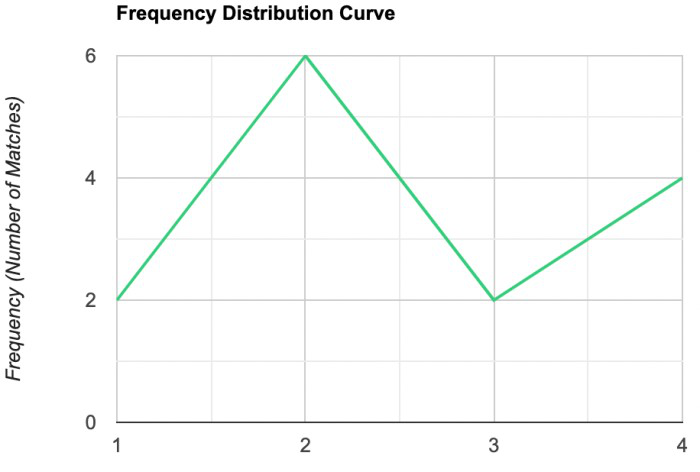Question 4: The table below gives the values of temperature recorded in Hyderabad for 25 days in summer. Represent the data in the form of less than type cumulative frequency distribution:

Solution:

Since there are so many distinct values here, we will use grouped frequency distribution. Let’s say the intervals are 20-25, 25-30, 30-35. Frequency distribution table can be made by counting the number of values lying in these intervals.

This is the grouped frequency distribution table. It can be converted into cumulative frequency distribution by adding the previous values.

The table above is the cumulative frequency distribution of the above data. Now let’s represent this in the form line curve for cumulative frequency distribution.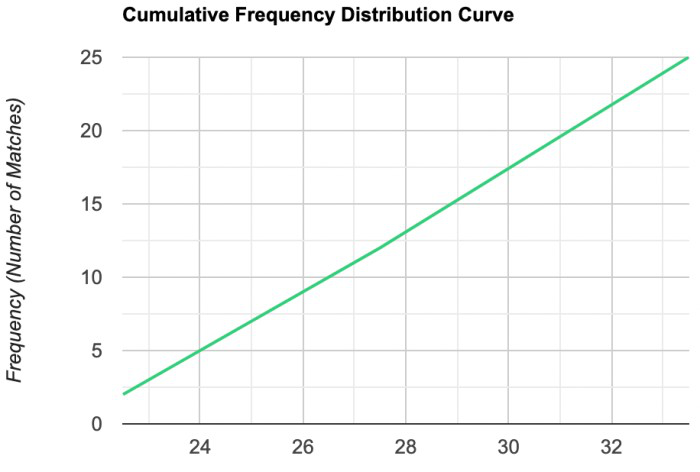My Personal Notes arrow_drop_up
Related Articles